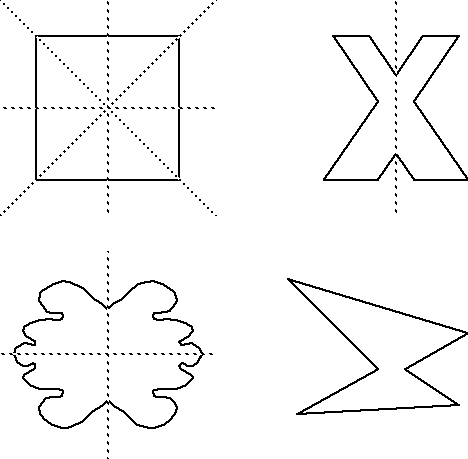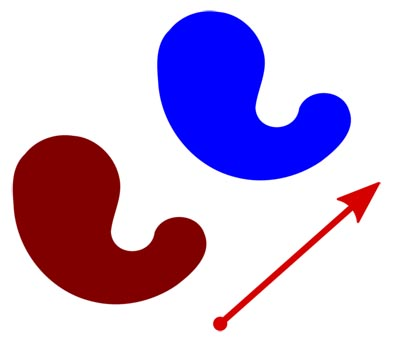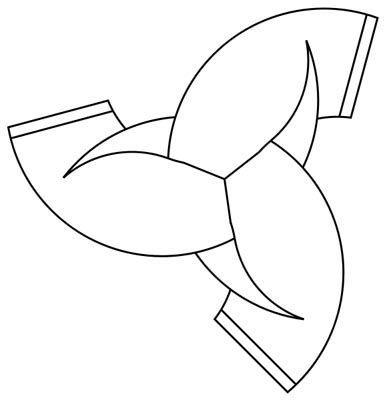Understanding Translations, Reflections, and Rotations in Geometry

# Understanding Translations, Reflections, and Rotations in Geometry

After a basic understanding of shapes has begun, it is imperative to consider the many different ways in which these shapes can then be moved throughout space while still retaining their original characteristics. Understanding that shapes which have been moved do not necessarily become new shapes, but remain constant in a new position on the plane they have been placed is important. It is through this skill process that one can identify which shapes are indeed different and new and which shapes have simply been moved to a new position in space. The process of transforming shapes is a part of symmetry.
Want to learn more? Take an online course in Geometry.

When considering shapes in spaces, it is imperative to address the movements of shapes in various ways. Typically, there are considered to be three ways in which shapes can be moved. These ways are: flipped, slid, or turned. The shape before it is moved in anyway becomes known as the preimage. The preimage becomes the image once the move occurs. Both the preimage and the image are identical in all aspects except for the coordinates within space they exist.

Any movement of an object can be referred to as a transformation. As each point of the object is considered individually, the transformation maps each point individually and tracks how each is moved or changed. In the case of most transformations, the points of the object are tracked or mapped based on the plane itself and all of the lengths will remain constant. Isometries is a term used to refer to these types of transformations. There are four types of isometries which are: translations, rotations, reflections and glide reflections. There are other types of translations, called dilatations, which do not maintain the various lengths.When a shape is flipped over a line, it is known as a reflection. Reflections are best understood when considering a mirror and how objects appear flipped when viewed in the mirror. The line, over which the shape is flipped, becomes known as the line of reflection. In reflections, it is important to remember that the shapes always keep the same size and shape, but the images are opposite of the original image (reversed). In this way, a reflection is a mirror image of the shape provided. Your own reflection as you look into the mirror is an example of this process of reflection.

Tessellations can demonstrate reflective symmetry. A tessellation can be said to have reflective symmetry if there is a point in the tessellation where a reflection of the original tessellation can be seen. The line where this reflection occurs is known as the mirror line.When a shape is slid to a new location in space, it is called a translation. Translations are what you would do if you slid a shape across a table to your partner. In considering the points of the object, all of them move in the same direction for the same distance. It does not affect the order of the points around the object. In order for a movement to be a true translation, the object must be moved with no reflecting or rotation of the object. Translations can occur in a horizontal nature (across a straight horizontal line), a vertical nature (across a straight vertical line), or in a diagonal nature (across a straight diagonal line). In a translation, all parts of the shape are sliding in the same direction and continue to move at the same rate (cover the same amount of space). When an object has been translated, it must be moved in a specific direction across a specific distance. Therefore, all translations have some direction and distance which can be described and measured.
All tessellations demonstrate the concept of translation. It could be said that all tessellations are repeated patterns and all translations will create some sort of repeated pattern. Therefore, it can be logically deduced that all tessellations have translational symmetry.When a shape is turned, it is called a rotation. A rotation of a shape occurs around one point. The shape is moved the point by any number of degrees. Since it occurs around one point, every rotation has a center and an angle. The rotation can occur in either a clockwise or counterclockwise direction. Therefore, every rotation can be described in terms of the angle by which the rotation occurred. When an object is rotated one hundred eighty degrees or half way around it is referred to as a half turn. When an object is rotated ninety degrees or one fourth of a turn, it is referred to as a quarter turn. In considering rotations, the number of matches the object will make as it completes one rotation is known as the order. There cannot be an order of one, as if the object requires one full rotation to align; it really does not show rotational symmetry.

In order for a tessellation to be considered as having rotational symmetry it must represent the exactly same tessellation as the original after it has been rotated. If the tessellation does in fact show the same result as the original after it has been rotated, then it can be considered to be a tessellation with rotational symmetry.

Shapes can be moved in multiple ways at one time. Objects could be slid and reflected. A glide reflection happens when a reflection is combined with a translation. In the glide reflection, the translation must occur along the line of reflection or mirror line. The glide reflection is the only type of symmetry which involves more than one step.

In order for a tessellation to demonstrate glide reflective symmetry, the tessellation after the glide reflection occurs must show the same result as the original tessellation before the glide reflection. Any tessellation which shows both reflective symmetry and translational symmetry will automatically have glide reflectional symmetry. However, all tessellations which have glide reflective symmetry do not necessarily have reflective symmetry or translational symmetry.

When recording any form of transformation, one will utilize the coordinate system. In the coordinate system, ordered pairs are used to represent points on a graph. When looking at a graph, the horizontal axis is referred to as the x axis. In a set of ordered pairs, the first number in the ordered pair refers to the x point. This tells you how many spaces to move horizontally. The vertical axis is referred to as the y axis. In ordered pairs, the second number refers to the y point. This tells you how many spaces to move vertically. Therefore, the ordered pair (6, 5) would tell you to move horizontally (over) six places and then up (vertically) five places. The space you come to on the graph is the spot represented by the given ordered pair. It is through the use of ordered pairs that one can describe the placement of objects on a plane and later, how they have been transformed.

Explore